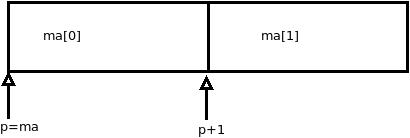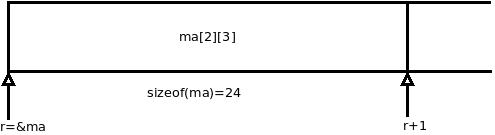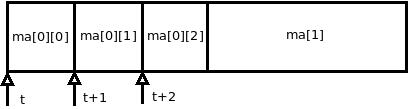# 多维数组那回事儿

2011年2月10日 由 edsionte

### 多维数组

```#include < stdio.h >

int main()
{
int ma;
int (*r);
int (*p);
int *t;

/*代码段1*/
p = ma;
printf("sizeof(ma)=%d\n",sizeof(ma));
printf("ma      =%p\tp   =%p\n",ma,p);
printf("p+1 =%p\n",p+1);
/*代码段2*/
r = &ma;
printf("sizeof(ma)=%d\n",sizeof(ma));
printf("&ma     =%p\tr  =%p\n",&ma,r);
printf("&ma+1   =%p\tr+1=%p\n",&ma+1,r+1);
/*代码段3*/
t = ma;
printf("sizeof(ma)=%d\n",sizeof(ma));
printf("ma   =%p\tt   =%p\n",ma,t);
printf("ma+1 =%p\tt+1 =%p\n",ma+1,t+1);
return 0;
}```ma和ma都是一个长度为3的整型数组，现在以ma为例进行说明。ma中包含三个元素ma，ma和ma。在代码段3中将ma赋值给t，则t指向数组ma的第一个元素a，则t+1和t+2则依次指向第二个元素和第三个元素。```edsionte@edsionte-laptop:~/code/expertC\$ gcc array.c -o array
edsionte@edsionte-laptop:~/code/expertC\$ ./array
sizeof(ma)=12
ma   =0xbfdfaa6c	p=0xbfdfaa6c
p+1  =0xbfdfaa78
sizeof(ma)=24
&ma  =0xbfdfaa6c	r=0xbfdfaa6c
r+1  =0xbfdfaa84
sizeof(ma)=4
ma=0xbfdfaa6c	t=0xbfdfaa6c
t+1  =0xbfdfaa70```

### 多维数组的初始化

```#include < stdio.h >

int main()
{
int m[] = {1,2,3};
int n[] = {1,2,3,};

printf("length(m)=%d\n",sizeof(m)/sizeof(m));
printf("length(n)=%d\n",sizeof(n)/sizeof(n));
return 0;
}

/* 编译并运行 */
edsionte@edsionte-laptop:~/code/expertC\$ gcc init_array.c -o init_array
edsionte@edsionte-laptop:~/code/expertC\$ ./init_array
length(m)=3
length(n)=3```

```#include < stdio.h >

int main()
{
int b[] = {1,2,1,1};
int c[] = {{1,2,1},{1,2,3},};

printf("length(b)=%d\n",sizeof(b)/sizeof(b));
printf("length(c)=%d\n",sizeof(c)/sizeof(c));
return 0;
}

/* 编译并运行 */
edsionte@edsionte-laptop:~/code/expertC\$ gcc init_array.c -o init_array
edsionte@edsionte-laptop:~/code/expertC\$ ./init_array
length(b)=2
length(c)=2```

### 它总是迷惑你！

```	char ma={
{{1,2},{2,3},{3,4}},
{{3,5},{4,5},{3,3}}
};

sizeof(ma[0,1,1])=？```

```		char ma = {
(1,2),(3,4),(5,3)
};

ma=？```

《C专家编程》 人民邮电出版社；（美）林登（LinDen.P.V.D） 著，徐波 译；